Closescroll

# Regression vs Classification in Machine Learning

The two most classic machine learning types, regression, and classification are still widely used in various application areas. Regression is used to determine the output predicted value from the input parameters. Linear and logistic regression are the most common types of regression used to solve practical problems.

Classification is used to determine whether an entity with certain input parameters belongs to a class within the existing characteristics of the input values. Both regression and classification are very often and effectively applied in machine learning, including for economic problems, marketing analysis, weather forecasting, social behavior calculations, observation of complex technical systems, and so on.

Let's look at regression and classification with simple examples and make test scripts in Python.

## What is machine learning?

Machine learning is a branch of artificial intelligence in computer science that often uses statistical techniques to give computers the ability to "learn", gradually improve performance in a given task from data without being explicitly programmed.

The name machine learning was initially used in 1959 by Arthur Lee Samuel. It evolved from research into pattern recognition and the theory of computational learning in the field of artificial intelligence. Machine learning explores the study and construction of algorithms that can learn and make predictions from data.

Machine learning algorithms overcome the adherence to strictly static software instructions, making data-driven predictions or decision-making by building a model of sample inputs. Machine learning is used in a number of computational problems in which the development and programming of explicit algorithms with good performance are difficult or impossible. Examples of applications include e-mail filtering, detection of network intruders or malicious insiders, optical character recognition (OСR), ranking training and computer vision, and economic process forecasting tasks.

Mathematical optimization provides the field of machine learning with methods, theory, and applied areas. Machine learning is used to teach and establish the basic characters of the behavior of various subjects and then used to find clear anomalies. Within the field of data analysis, machine learning is a method used to invent complex models and algorithms that serve for forecasting. In commercial applications, this is known as predictive analytics.

## Regression basics

Regression is a technique used to model and analyze relationships between variables. Regression is very useful when creating simple relationships and with relatively small amounts of data. Regression notation is intuitive and this kind of analysis is sensitive to outliers in the data.

One-dimensional, or simple linear regression, is a technique used to model the relationship between one independent input variable, i.e. the function variable, and the output dependent variable. The model is linear.

A more general case is multiple linear regression, where a model of the relationship between multiple input variables and an output dependent variable is created. The model remains linear because the output value is a linear combination of the input values. Also worth mentioning is polynomial regression. The model becomes a nonlinear combination of input variables, that is, there may be exponential variables among them: sine, cosine, etc.

The regression model can be represented as: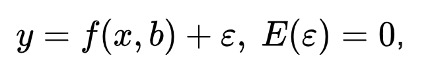where b - model parameters, ε - random model error; it is called linear regression if the regression function f (x, b) has the form:where bj are regression parameters (coefficients), xj are regressors (model factors), k is the number of model factors.

There are the following basic regression options:

• linear regression
• multiple linear regression
• polynomial regression
• ridge regression
• lasso regression
• elastic net regression

Examples of regressions are problems such as the organization's cost model (without indicating a random error) and the simplest consumer spending model (Keynes).

## Classification basics

Classification (Latin “classis” - class and “facio” - do) - a system of distribution of objects in groups according to predefined features. In some cases, the term categorization is used to mean the division of objects into categories.

Classification is one of the parts of machine learning, dedicated to solving the following problem. There are many objects that are divided into classes in some way. A finite set of objects with known classes is specified. This set is called the training sample. The class affiliation of the other objects is unknown. The task is to build an algorithm that can classify an arbitrary object from the original set.

To classify an object means to specify the name of the class to which the object belongs. Object classification is a class number or name issued by a classification algorithm as a result of its application to a particular object.

As a formal statement of the problem, we can consider X as a set of descriptions of objects, Y - a finite set of numbers (names, labels) of classes. There is an unknown target dependence - mapping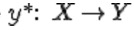, the values of which are known only on the objects of the final training sample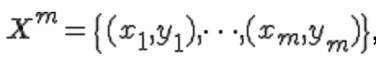We need to build an algorithm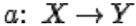that can classify an arbitrary object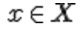.

There are the following main classification options:

• Binary Classification
• Multi-Class Classification
• Multi-Label Classification
• Imbalanced Classification

## Example of regression and classification

Let's start with a simple regression example. In this example of regression, we have two variables as input. And at the output, we have one variable, which depends on the input. We are doing linear regression using LinearRegression() function from sklearn library from https://scikit-learn.org/. That is, the output values will be averaged using a straight line that depends on the set of training values. Then using reg.predict(), the output y value can be calculated very easily from the set of input values. Thus, it is possible to predict the values of the input variables even in the case when it is difficult to build a direct relationship.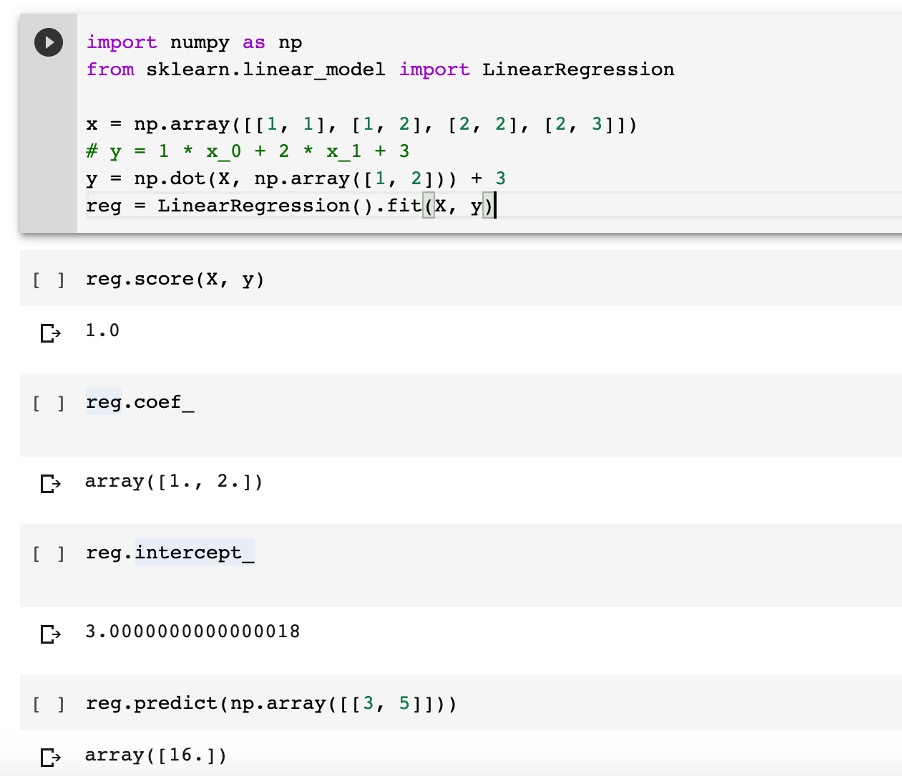Now let's look at a simple example of classification. This example uses a diabetes detection dataset, a classic example for machine learning. Using a set of input parameters, we determine whether a person has diabetes or not. Dataset is included to sklearn library

Decision Tree Classifier is used for training.Now let's run this script and execute: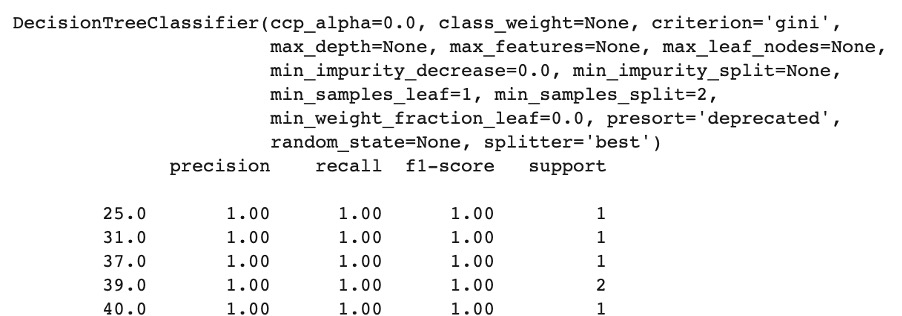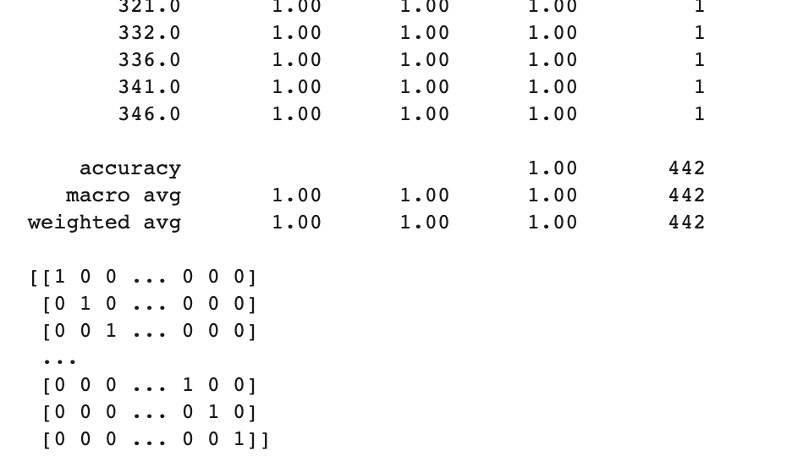First, we train the model, then we input the test data which was not in the training dataset. Then we check l that the input dataset really coincides with the output results, that is, the objects were correctly assigned to the selected class (i.e. diabetes or no diabetes).

Let's consider now the similarities and differences between regression and classification:

• regression and classification are powerful machine learning tools, they allow you to analyze data when it is very difficult to deduce the dependence between the input and output data explicitly
• regression allows you to predict the value of the output from a set of input data for a pretrained dataset
• classification allows you to identify which group a given entity belongs to based on the input dataset for a pretrained dataset

Fig. 1. An example of classification and comparison with regression.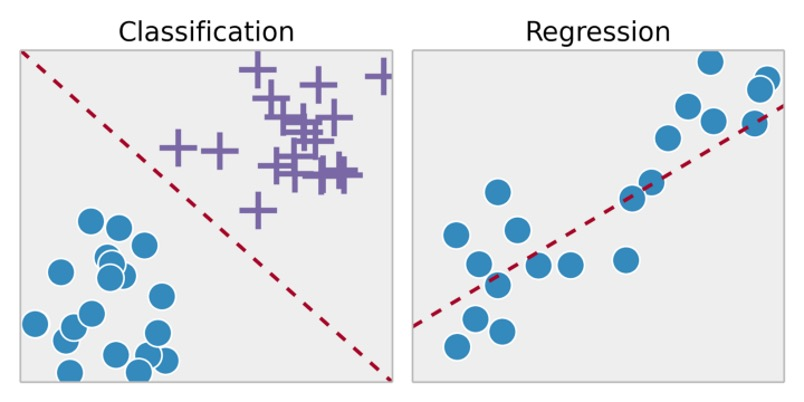## Conclusion

In conclusion, we note that such seemingly simple machine learning methods allow solving a huge class of problems. Their combinations generally give very good results in data analysis. For more details, please read this great article.

Our experts from Svitla Systems will carry out your machine learning projects. We have a wide range of knowledge and a good mathematical background. Many of our data scientists and machine learning specialists have PhDs in Computer Science or Mathematics.

We have experience in conducting large projects in the field of machine learning, neural networks, and software development. Our experience in the development of combined projects, information systems with machine learning elements, is especially valuable. Such projects are especially useful and are of great benefit in solving real problems.

by Svitla Team
September 10, 2020